American Journal of Optics and Photonics
Volume 2, Issue 6, December 2014, Pages: 69-74

Electrical and photoelectrical properties of copper(II) complex/n-Si/Au heterojunction diode

Cihat Ozaydin1, Kemal Akkilic2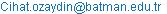(C. Ozaydin)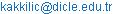(K. Akkilic)

Cihat Ozaydin, Kemal Akkilic. Electrical and Photoelectrical Properties of Copper (II) Complex/n-Si/Au Heterojunction Diode. American Journal of Optics and Photonics. Special Issue: Organic Photonics & Electronics. Vol. 2, No. 6, 2014, pp. 69-74. doi: 10.11648/j.ajop.20140206.11

Abstract: In this study, we fabricated copper(II) complex/n-Si/Au organic-inorganic heterojunction diode by forming copper(II) complex thin film on n-type silicon. A direct optical band gap energy values of the copper(II) complex (Cu2C34H34N2O21Cl4) thin film on a glass substrate was obtained as Eg=2.98 eV. The current-voltage (I-V) measurement of the diode was carried out at room temperature and under dark. The ideality factor n and barrier height ϕb values of the diode were found to be 3.17 and 0.71 eV, respectively. The diode indicates non-ideal current-voltage characteristics due to the high ideality factor greater than unity. The series resistance Rs and ideality factor n values were determined using Cheung’s method and obtained as 5.54 kΩ and 3.81, respectively. The capacitance-voltage (C-V) measurements of the diode were performed at different frequency and room temperature. From the analysis of the C-V measurements carrier concentration Nd, diffusion potential Vd and barrier height values ϕbc-v were determined as 2.79x1015 cm-3, 1.078 V, 1.31 eV, respectively. From the I-V measurements of the diode under 1.5 AM illumination, short circuit current (Isc) and open circuit voltage (Voc) have been extracted as 12.8 µA and 153 mV, respectively.

Keywords: Organic-İnorganic, Heterojunction, Schottky Contact, Photovoltaic Properties

1. Introduction

Organic materials have received increasing attraction as they possess interesting properties compared with the conventional inorganic semiconductors. Recent extensive studies have shown that organic materials exhibit a variety of interesting optical, electrical, and photoelectric properties in solid state physics . Organic materials have been chosen in the fabrication of devices because of their some advantages such as low cost and easy preparation techniques, possibility of synthesis for different purposes and large area coverage . The main advantages of these compounds may be chemical adjustability of their band gap and transport properties. So it is very important to find suitable organic compounds to fabricate electronic and optoelectronic devices based on organic materials. There are a number of ways to use organic molecules in the fabrication of electronic and optoelectronic devices. Organic–inorganic (OI) structure is one of them . Many authors have fabricated this kind of structures to benefit from advantages of both organic and inorganic materials in a single device and modify electrical and photoelectrical properties of metal–semiconductor (MS) devices [4–7]. OI structures have been used as solar cells [8–10], light emitting diodes [11–12], Schottky diodes [13–15], etc. Organic/ inorganic semiconductor heterojunctions investigated both due to the unusual nature of these contacts as well as to the potential new devices applications. Organic semiconductors exhibit rectification behavior when deposited onto inorganic semiconductor substrates .

In this study, we have used recently synthesized  binuclear copper(II) complex with [Cu2(L)(ClO4)2][ClO4]2 (L is C34H34N2O5) molecular formula as an organic material due to being rich conjugated electrons.  The molecular structure of the copper(II) complex is shown in Fig. 1. Our aim is to fabricate a copper(II) complex/n-Si/Au OI structure to observe whether or not it can be used as a rectifying contact and to determine the electrical and photoelectrical properties of the device by investigating current–voltage  under dark an illumination. To discuss how the diffusion potential is generated we measured the capacitance–voltage characteristics.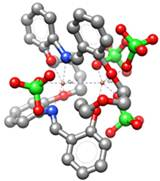Figure 1. The molecular structure of the copper(II) complex

2. Experimental Details

In this study, we used n-type Si wafer with  orientation, 400 μm and 1-10 Ωcm resistivity as a substrate. Firstly, n-si wafer was chemically cleaned by using RCA cleaning procedure (i.e. 10 min of boiling in NH3+H2O2+6 H2O followed by 10 min in HCl+ H2O2+6 H2O at60 oC). Then, a low resistivity ohmic contact was made by evaporating AuSb (99-1%), followed by a annealing treatment at 450oC for 3 min in N2 atmosphere. Before forming the organic layer on the substrate, the native oxide on front of surface n-Si substrate was removed in HF:H2O (1:10) solution for 30 s and finally the wafer was rinsed in deionized (DI) water. The copper(II) complex was dissolved in dimethylformamide and it coated on to the clean n-Si substrate using a spin coater at 1500rpm for 30s. After coating, the film was allowed to dry by itself for one day. To perform electrical measurements, Au was evaporated onto the copper(II) complex layer at 2x10–6 Torr (diode area1.76x10–2 cm2). The experimental setup of the copper(II) complex/n-Si/Au heterojunction diode is shown in Fig. 2.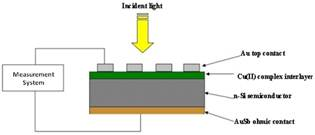Figure 2. The experimental setup of the Copper(II) complex/n-Si/Au heterojunction diode for electrical and photoelectrical characterization.

I–V and C–V measurements were performed using a Keithley 4200-SCS instrument and a HP4294A impedance analyzer, respectively, at room temperature. Photovoltaic measurements were employed using solar simulator under 1.5 AM illumination. Also, optical spectrum of the copper (II) complex thin film on a glass substrate was taken with Perkin Elmer Lambda 25 UV-Vis spectrometer.

3. Results and Discussion

3.1. Optical Properties of Copper(II) Complex Thin Film

The optical absorption spectrum of the copper(II) complex thin film on glass substrate is given in the inset of Fig. 3. Analysis of the optical absorption spectra can be used for the investigation of the optically induced electronic transitions in terms of band to band transition theory, and can provide essential information about the optical energy band gap of semiconductor materials. The energy gaps, Eg, and type of electronic transitions were determined from the following relationship: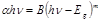(1)

Where B is a constant, Eg is the optical band gap, α is absorption coefficient of the film, h  is the plank constant and m is equal to m=1/2, 2, 3/2 or 3 for allowed direct, allowed non-direct, forbidden direct or forbidden non-direct transitions, respectively.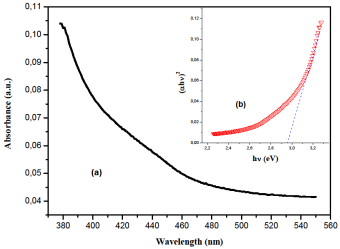Figure 3. plot of (αhυ)2 vs. hυ of the Copper(II) complex thin film

Fig. 3 shows plot of (αhυ)2 vs. of the copper(II) complex thin film according to Eq. (1). Satisfactory fit is obtained for (αhυ)2 vs. indicating the presence of a direct band gap. The optical energy band gap Eg of the organic thin film was determined as 2.98 eV by extrapolating the linear portion of this plot at (αhυ)2=0, which indicates that direct allowed transitions dominates in the copper(II) complex thin film.

3.2. Current–Voltage Properties of Copper(II) Complex/n-Type Silicon/au Heterojunction under Dark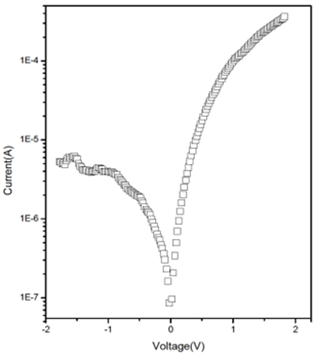Figure 4. Experimental semi-log I– V characteristic of the Copper(II) complex/n-Si/Au heterojunction

Fig. 4 shows the experimental semi-log I– V characteristic of the copper (II) complex/n-Si/Au heterojunction diode under dark at room temperature.

The dark I–V characteristics indicate that the diode exhibits a rectifying behavior. Therefore, it can be assumed that the device behaves as a Schottky diode and the thermionic emission (TE) theory can be used to obtain electrical parameters of the device. According to the theory, the dark I–V characteristics of Schottky contacts for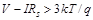can be expressed as [18,19]: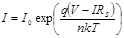(2)

Where V is the applied voltage, n is the dimensionless ideality factor, RS is the series resistance and I0 is the saturation current expressed as: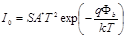(3)

Where S is the diode area, A* is the effective Richardson constant (112A/cm2K2for n-Si) , k is the Boltzmann constant, T is absolute temperature, q is the electron charge and Φb is the barrier height. From Eqs. (2) and (3), ideality factor n and barrier height Φb can be written as: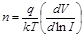(4)

and,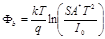(5)

Using the TE theory , the ideality factors n and Φb for the heterojunction diode have been obtained from the slopes and the current axis intercepts of the linear regions of the corresponding forward bias I–V plot, respectively. The value of ideality factor and barrier height was obtained as n=3.17 and Φb=0.71eV. For an ideal diode n=1, nevertheless the ideality factor n has usually greater than unity. The diode indicated a non-ideal current–voltage behavior due to the ideality factor being higher than unity. The higher values of n could be attributed to the presence of a wide distribution of low schottky barrier height patches caused by lateral barrier inhomogeneities. Also, the image-force effect, recombination-generation, and tunneling may be possible mechanisms that could lead to the ideality factor value greater than unity . The calculated barrier height of the studied diode is lower than barrier height (0.79) of conventional Au/n-Si schottky diode previously reported in literature . This case may be ascribed to the copper(II) complex interlayer modifying the effective barrier height by influencing the space charge region of the inorganic silicon substrate. The organic layer can produce substantial shift in the work function of the metal and in the electron affinity of the semiconductor by giving an excess for the barrier height, i.e., the barrier height of Au/n-Si diode decreases by the insertion of organic layer between n-Si semiconductor and metal . In literature, barrier height modifications have been reported experimentally by insertion of organic thin films between the metals and the semiconductors. The studies have shown that effective Schottky barrier could be either increased or decreased by using organic thin layer on inorganic semiconductor For example, Cakar et al.  have formed Cu/pyronine–B/p–Si, Au/ pyronine–B/p–Si, Al/pyronine–B/p–Si and Sn/pyronine–B/p–Si diodes and calculated the effective barrier heights of diodes larger than the conventional metal/p–Si contacts. Farag et al.  obtained a BH value of 0.767eV for Au/OXZ/n-Si/Al heterojunction contact and they have shown that the effect of oxazine (OXZ) layer decreases the barrier height of the conventional Au/n-Si diode. When the applied voltage is sufficiently large, the effect of the RS can be seen at the non-linear regions of the forward bias I–V characteristics. The series resistance RS, as well as other Schottky diode parameters such as ideality factor (n) and BH was also determined using the Cheung's functions . According to Cheung and Cheung, the forward bias I–V characteristics due to the TE of the Schottky diode with the series resistance can be expressed as: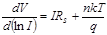(6)(7)

and H(I) is given as follows: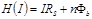(8)

Fig. 5 shows the plots of dV/d ln I–I and H(I)–I obtained by using the Cheung’s functions given in Eqs. (6) and (8). The values of series resistance RS and ideality factor n were found to be 5.54 kΩ and 3.81 from dV/d ln I–I plot, respectively. Also, the value barrier height Φb were found to be 0.69 eV from H(I)–I plot, respectively. There is relatively a difference between the values of ideality factor obtained from the downward curvature regions of forward bias I-V plots and from the linear regions of the same characteristics. This could be attributed to the existence of effects such as series resistance and the bias dependence of SBH according to the voltage drop across the interfacial layer and charge of the interface states with the bias in the low voltage region of the I-V plot .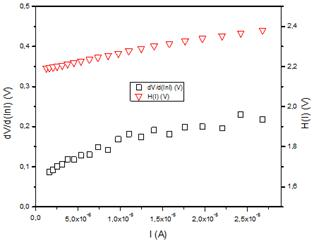Figure 5. plots of dV/d ln I–I and H(I)–I for the Copper(II) complex/n-Si/Au heterojunction diode

3.3. Capacitance–Voltage Characteristics of Copper(II) Complex/n-Si/Au Heterojunction Diode

For metal/semiconductor devices, capacitance measurements are an important nondestructive method for obtaining information about the fixed charge concentration and the barrier height. Fig. 6 shows the capacitance–voltage characteristics of the copper (II) complex/n-Si/Au diode under various frequencies at room temperature.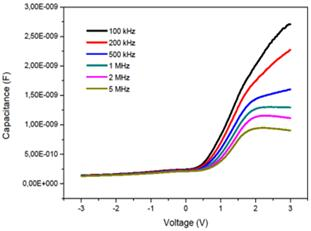Figure 6. Capacitance–voltage characteristics of the Copper(II) complex/n-Si/Au diode under various frequencies

As seen in Fig. 6, at positive voltages the capacitance decreases with increasing frequency due to the movements of interface charges in the depletion layer of the diode. As shown in Fig. 6, if the CV measurement carries out at sufficiently high frequencies, the charge at the interface cannot follow an a.c. signal. In Schottky diodes, the depletion layer capacitance can be expressed as ;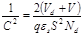(9)

where S is the area of the diode, Vd is the diffusion potential at zero bias and is determined from the extrapolation of the linear C2–V plot to the V axis at 500 kHz. shown in Fig. 7.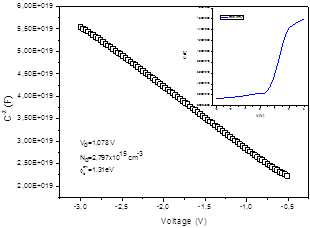Figure 7. C–2–V Plot of the Copper(II) complex/n-Si/Au diode at 500 kHz

The value of the barrier height Φb can be calculated by using the C–V measurements by the following well-known equation: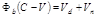(10)

where Vn is the potential difference between the Fermi level and the conduction band minimum for n-Si. It can be calculated using the known carrier concentration Nd  according to;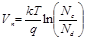(11)

where Nc=2.8x1019 cm–3 is the density of effective states in the conduction band of n-Si . Fig. 7 shows C–2–V plots of the Copper(II) complex/n-Si/Au MIS capacitor  at 500 kHz. Values for Vd  and  Nd can be obtained from the intercept and slope of a C–2–V plot (Fig. 7). We calculated values of Vd=1.078 V, Φb=1.31 eV and Nd=2.79x1015 cm-3. As seen from the above values, the difference between Φb (I–V) and Φb (C–V) for Au/Cu(II)complex/n-Si OI devices originates from the different nature of the I– V and C– V measurements. Due to different nature of the C– V and I– V measurement techniques, barrier heights deduced from them are not always the same. The capacitance C is insensitive to potential fluctuations on a length scale of less than the space charge region and C– V method averages over the whole area and measures to the described BH. (Φb (C–V) is a mean value of the barrier height distribution) The DC current I across the interface depends exponentially on barrier height and thus sensitively on the detailed distribution at the interface (Φb (I–V) is a minimum value of the barrier height distribution) . In this regard, the barrier height values deduced from I-V measurements is expected to be lower than those determined from C-V measurements.

3.4. Photoelectrical Properties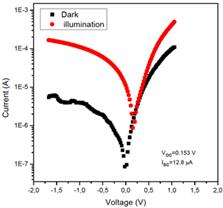Figure 8. Current–voltage characteristics of the Copper(II) complex/n-Si/Au diode under dark and illumination conditions.

The current–voltage (IV) characteristics of Au/Cu(II)complex/n-Si diode under dark and light illumination conditions are shown in Fig. 8. It can be seen that the current value at a given voltage for the Au/Cu(II)complex/n-Si under illumination is higher than that in dark. This indicates that the light illumination increases production of electron–hole pairs. This suggests that the diode exhibits a photovoltaic behavior. In this device, the carrier charges are generated when the copper(II) complex absorbs light and are driven towards the contacts. The generated charges produce a photovoltage at open circuit and a photocurrent at short circuit. The reverse current increases the efficient substantially due to charge separation near the interface of the device . The increase in charge production is dependent on electrical properties of the device. The device shows a photovoltaic behavior with a maximum open-circuit voltage (Voc) of 153 mV and a short-circuit current (Isc) of 12.8 µA under 100 mW/cm2 and AM1.5 illumination condition.

4. Conclusion

Electrical and photovoltaic properties of the copper(II) complex/n-Si heterojunction have been investigated. A direct optical band gap energy values of the copper(II) complex thin film was obtained as Eg=2.98 eV absorption and transmittance spectra. The ideality factor n and barrier height ϕb values of the diode in dark were found to be 3.17 and 0.71 eV, respectively. By using the capacitance–voltage measurement, the barrier height and free carrier concentration values for the copper(II) complex/n-Si diode have been calculated as 1.31 eV and 2.79x1015 cm-3, respectively. Series resistance calculation has also been made by using Cheung functions. It has been observed that the photocurrent in the device in reverse direction is strongly enhanced by photo illumination and the device shows photovoltaic properties with electronic parameters, a maximum open-circuit voltage of 153 mV and a short-circuit current of 12.8 µA under 1.5 AM illumination. The obtained results have suggested that copper(II) complex  can be used in the fabrication of electrical and photoelectrical devices based on organic compounds.

Acknowledgements

The Authors wish to thank Assoc. Prof. Dr. Salih İlhan from Siirt University due to organic material (Copper(II) complex) supply.

References

1. M.M. El-Nahass, H.S. Metwally, H.E.A. El-Sayed, A.M. Hassanien, Materials Chemistry and Physics 133 (2012) 649– 654.
2. K. Akkılıç, Y.S. Ocak, T. Kılıcoğlu, S. İlhan, H. Temel, Current App. Physics 10 (2010) 337–341.
3. T. Kılıcoğlu, Y.S. Ocak, Microelectronic Engineering 88 (2011) 150–154.
4. A.R. Vearey–Roberts, D.A. Evans, Appl. Phys. Lett. 86 (2005) 072105.
5. A.A.M. Farag, W.G. Osiris, I.S. Yahia, Synthetic Metals, Volume 161, Issues 17–18, September 2011, Pages 1805-1812.
6. R.K. Gupta, K. Ghosh, P.K. Kahol, Physica E 41 (2009) 1832.
7. F. Yakuphanoglu, B.F. Senkal, Synth. Met. 159 (2009) 311.
8. B. O’Regan, M. Gratzel, Nature 353 (1991) 737.
9. Ö. Güllü, A.Türüt, Sol.Energy Mater. Sol. Cells 92 (2008) 1205.
10. M.M. El-Nahass, H.M. Zeyada, K.F. Abd-El-Rahman, A.A.A. Darwish, Sol. Energy Mater. Sol. Cells 91 (2008) 1120.
11. N.D. Kumar, M.P. Joshi, C.S. Friend, P.N. Prasad, R. Burzynski, Appl. Phys. Lett. 71 (1997) 1388.
12. S. Guha, R.A. Haight, N.A. Bojarczuk, D.W. Kisker, J. Appl. Phys. 82 (1997) 4126.
13. R.K. Gupta, R.A. Singh, J. Polym. Res. 11 (2004) 269.
14. A. Ashery, A.A.M. Farag, M.A. Salem, Microelectron. Eng. 85 (2008) 2309.
15. T. Kılıcoğlu, Thin Solid Films 516 (2008) 967.
16. Kemal Akkılıç, Fahrettin Yakuphanoglu, Microelectronic Engineering 85 (2008) 1826–1830.
17. S. İlhan. Journal of Coordination Chemistry 61 (2008) 2884–2895.
18. Sze S M and Kwok K Ng 2007 Physics of SemiconductorDevices 3rd edn (NJ: Wiley)
19. E. H. Rhoderick and R. H. Williams, Metal-Semiconductor Contacts 2nd ed. (Clarendon, Oxford, 1988).
20. R.T. Tung, Phys. Rev. B 45 (23) (1992) 13509.
21. M. Sağlam, F.E. Cimilli, A. Türüt, Physica B 348 (2004) 397–403.
22. M. Cakar, C. Temirci, A. Turut, Synth. Met. 142 (2004) 177.
23. A.A.M. Farag, E.A.A. El–Shazly, M. Abdel Rafea, A. Ibrahim, Sol. Energy Mater. Sol. Cells 93 (2009) 1853-1859.
24. S.K. Cheung, N.W. Cheung, Appl. Phys. Lett. 49 (1986) 85.
25. P. Chattopadhyay, Solid-State Electron. 37 (1994) 1759.
26. F. Yakuphanoglu, Solar Energy Mater, Solar Cells 91 (13) (2007) 1182.

 Contents 1. 2. 3. 3.1. 3.2. 3.3. 3.4. 4.
Article ToolsAbstractPDF(349K)